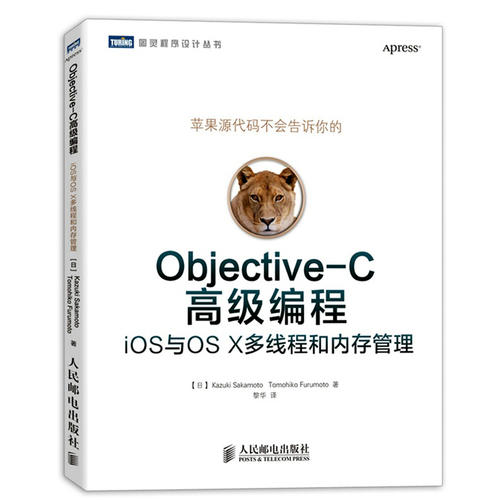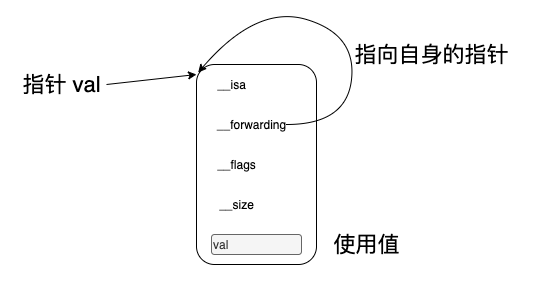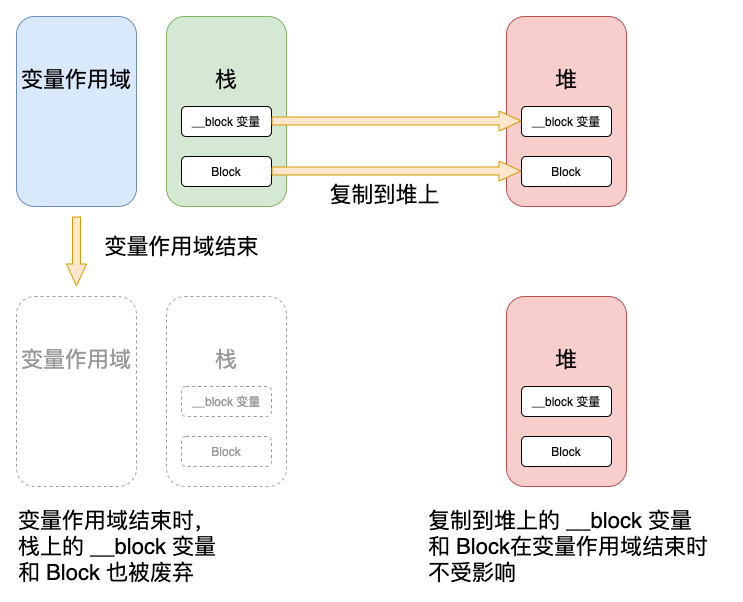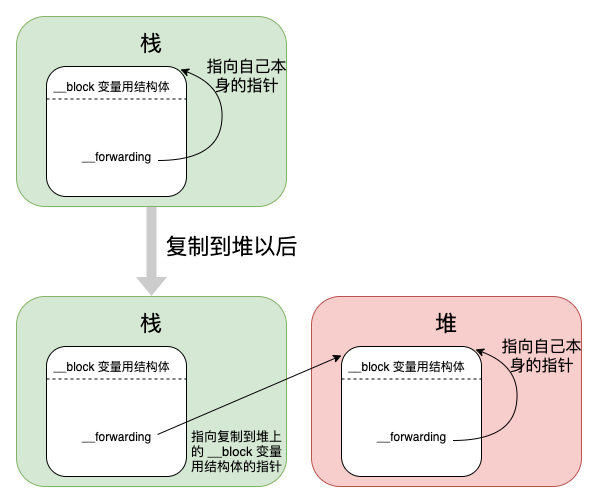# Blocks## 什么是Blocks

`Block``C` 语言的扩充功能：带有自动变量的匿名函数。

• 自动变量： 局部变量
• 匿名函数： 不带有名称的函数

`C` 语言的标准库中不允许存在这样的函数。

• 使用函数名称调用函数
• 使用函数指针调用函数
``````// 函数声明
int func(int count);

// 使用函数名称调用函数
int result = func(10);

// 使用函数指针调用函数
int (*funcptr)(int) = &func;
int result = (*funcptr)(10);
``````
• `&` 取地址
• `*` 取值

C 语言的函数中可能使用的变量：

• 自动变量（局部变量）
• 函数的参数
• 静态变量（静态局部变量）
• 静态全局变量
• 全局变量

• 静态变量（静态局部变量）
• 静态全局变量
• 全局变量

C++ 和 Objective-C 使用类可以保持变量值且能够多次持有该变量自身。

## Blocks 模式

### Block 语法

• 没有函数名
• 带有 `^`

C 语言函数定义如下：

`返回值类型` `函数名称` `参数列表` `表达式`

Block 语法格式如下：

`^` `返回值类型` `参数列表` `表达式`

`^` `参数列表` `表达式`

`^` `表达式`

### Block 类型变量

``````int func(int count) {
return count + 1;
}

int (*funcptr)(int) = &func;
``````

``````int (^blk)(int);
``````

Block 类型的变量的用途：

• 自动变量
• 函数参数
• 静态变量
• 静态全局变量
• 全局变量

``````int (^blk)(int) = ^(int count) { return count + 1; };
``````

``````int (^blk1)(int) = blk;

int (^blk2)(int);
blk2 = blk;
``````

``````void func(int (^blk)(int)) {
}
``````

``````int (^func())(int) {
return ^(int count) { return count + 1; };
}
``````

``````typedef int (^blk_t)(int);

// 作为函数参数
void func1(blk_t blk) {
}

// 作为函数返回值
blk_t func2() {
return ^(int count) { return count + 1; };
}
``````

### 截获自动变量值

“带有自动变量值”在 Blocks 中表现为“截获自动变量值”。

``````void autoGetValueForBlock() {

int dmy = 256;
int val = 10;

const char *fmt = "val = %d\n";

void (^blk)(void) = ^ { printf(fmt, val); } ;

val = 2;

fmt = "These values were changed. val = %d\n";

blk();
}
``````

Block 语法的表达式使用的它之前声明的自动变量 `fmt``val`。 Blocks 中，Block 表达式所使用的自动变量的值，即保存该自动变量的瞬间值。 因为 Block 表达式保持了自动变量的值，所以在执行 Block 语法后，即使改写 Block 中使用的自动变量的值也不会影响执行时自动变量的值。

``````val = 10
``````

### __block 说明符

``````int val = 0;

void (^blk)(void) = ^{ val = 1; };

blk();

printf("val = %d\n", val);
``````

``````Variable is not assignable (missing __block type specifier)
``````

``````__block int val = 0;

void (^blk)(void) = ^{ val = 1; };

blk();

printf("val = %d\n", val);
``````

``````val = 1
``````

### 截获的自动变量

``````NSMutableArray *array = [[NSMutableArray alloc] init];

void (^blk)(void) = ^ {
id obj = [[NSObject alloc] init];
};
``````

## Blocks 的实现

### Block 的实质

Block 语法看上去好像很特别，但它实际上是作为极普通的 C 语言源代码来处理的。 通过支持 Block 的编译器，含有 Block 语法的源代码转换为一般 C 语言编译器能够处理的源代码，并作为极为普通的 C 语言源代码被编译。

clang（LLVM 编译器）具有转换为我们可读源代码的功能。 通过 `-rewrite-objc` 选项就能将含有 Block 语法的源代码变换为 C++ 的源代码。

``````clang -rewrite-objc 源代码文件名
``````

``````int main(int argc, const char * argv[]) {
void (^blk)(void) = ^{ printf("Block\n"); };

blk();

return 0;
}
``````

``````struct __block_impl {
void *isa;
int Flags;
int Reserved;
void *FuncPtr;
};

struct __main_block_impl_0 {
struct __block_impl impl;
struct __main_block_desc_0* Desc;
__main_block_impl_0(void *fp, struct __main_block_desc_0 *desc, int flags=0) {
impl.isa = &_NSConcreteStackBlock;
impl.Flags = flags;
impl.FuncPtr = fp;
Desc = desc;
}
};

static void __main_block_func_0(struct __main_block_impl_0 *__cself) {
printf("Block\n"); }

static struct __main_block_desc_0 {
size_t reserved;
size_t Block_size;
} __main_block_desc_0_DATA = { 0, sizeof(struct __main_block_impl_0)};

int main(int argc, const char * argv[]) {
void (*blk)(void) = ((void (*)())&__main_block_impl_0((void *)__main_block_func_0, &__main_block_desc_0_DATA));

((void (*)(__block_impl *))((__block_impl *)blk)->FuncPtr)((__block_impl *)blk);

return 0;
}
``````

### 截获自动变量值

``````int main(int argc, const char * argv[]) {
int dmy = 256;
int val = 10;

const char *fmt = "val = %d\n";

void (^blk)(void) = ^ { printf(fmt, val); } ;

blk();
return 0;
}
``````

``````struct __main_block_impl_0 {
struct __block_impl impl;
struct __main_block_desc_0* Desc;
const char *fmt;
int val;
__main_block_impl_0(void *fp, struct __main_block_desc_0 *desc, const char *_fmt, int _val, int flags=0) : fmt(_fmt), val(_val) {
impl.isa = &_NSConcreteStackBlock;
impl.Flags = flags;
impl.FuncPtr = fp;
Desc = desc;
}
};
static void __main_block_func_0(struct __main_block_impl_0 *__cself) {
const char *fmt = __cself->fmt; // bound by copy
int val = __cself->val; // bound by copy
printf(fmt, val); }

static struct __main_block_desc_0 {
size_t reserved;
size_t Block_size;
} __main_block_desc_0_DATA = { 0, sizeof(struct __main_block_impl_0)};
int main(int argc, const char * argv[]) {
int dmy = 256;
int val = 10;

const char *fmt = "val = %d\n";

void (*blk)(void) = ((void (*)())&__main_block_impl_0((void *)__main_block_func_0, &__main_block_desc_0_DATA, fmt, val)) ;

((void (*)(__block_impl *))((__block_impl *)blk)->FuncPtr)((__block_impl *)blk);
return 0;
}
``````

### __block 说明符

__block 存储域类说明符 （__block storage-class-specifier）

C 语言中有以下存储域类说明符：

• typedef
• extern
• static
• auto
• register

`__block` 说明符类似于 `static``auto``register` 说明符，它们用于指定将变量值设置到哪个存储域中。 例如： `auto` 表示作为自动变量存储在栈中， `static` 表示作为静态变量存储在数据区中。

``````int main(int argc, const char * argv[]) {
__block int val = 0;

void (^blk)(void) = ^{ val = 1; };

blk();

printf("val = %d\n", val);
return 0;
}
``````

``````struct __Block_byref_val_0 {
void *__isa;
__Block_byref_val_0 *__forwarding;
int __flags;
int __size;
int val;
};

struct __main_block_impl_0 {
struct __block_impl impl;
struct __main_block_desc_0* Desc;
__Block_byref_val_0 *val; // by ref
__main_block_impl_0(void *fp, struct __main_block_desc_0 *desc, __Block_byref_val_0 *_val, int flags=0) : val(_val->__forwarding) {
impl.isa = &_NSConcreteStackBlock;
impl.Flags = flags;
impl.FuncPtr = fp;
Desc = desc;
}
};
static void __main_block_func_0(struct __main_block_impl_0 *__cself) {
__Block_byref_val_0 *val = __cself->val; // bound by ref
(val->__forwarding->val) = 1; }
static void __main_block_copy_0(struct __main_block_impl_0*dst, struct __main_block_impl_0*src) {_Block_object_assign((void*)&dst->val, (void*)src->val, 8/*BLOCK_FIELD_IS_BYREF*/);}

static void __main_block_dispose_0(struct __main_block_impl_0*src) {_Block_object_dispose((void*)src->val, 8/*BLOCK_FIELD_IS_BYREF*/);}

static struct __main_block_desc_0 {
size_t reserved;
size_t Block_size;
void (*copy)(struct __main_block_impl_0*, struct __main_block_impl_0*);
void (*dispose)(struct __main_block_impl_0*);
} __main_block_desc_0_DATA = { 0, sizeof(struct __main_block_impl_0), __main_block_copy_0, __main_block_dispose_0};
int main(int argc, const char * argv[]) {
__attribute__((__blocks__(byref))) __Block_byref_val_0 val = {(void*)0,(__Block_byref_val_0 *)&val, 0, sizeof(__Block_byref_val_0), 0};

void (*blk)(void) = ((void (*)())&__main_block_impl_0((void *)__main_block_func_0, &__main_block_desc_0_DATA, (__Block_byref_val_0 *)&val, 570425344));

((void (*)(__block_impl *))((__block_impl *)blk)->FuncPtr)((__block_impl *)blk);

printf("val = %d\n", (val.__forwarding->val));
return 0;
}
``````

`__block` 变量也同 `Block` 一样变成 `__Block_byref_val_0` 结构体类型的自动变量，即栈上生成的 `__Block_byref_val_0` 结构体实例。

`__block` 变量赋值原理：

``````static void __main_block_func_0(struct __main_block_impl_0 *__cself) {
__Block_byref_val_0 *val = __cself->val; // bound by ref
(val->__forwarding->val) = 1; }
``````

`__Block_byref_val_0` 结构体实例变量 `__forwarding` 持有指向该实例自身的指针。 通过成员变量 `__forwarding` 访问成员变量 `val`### Block 存储域

• Block 转换为 Block 的结构体类型的自动变量
• __block 变量转换为 __block 变量的结构体类型的自动变量

Block 栈上 Block 的结构体实例
__block 变量 栈上 __block 变量的结构体实例

`Block` 也是 `Objective-C` 的对象。将 `Block` 当作 `Objective-C` 对象来看时，该 `Block` 的类为 `_NSConcreteStakBlock`

_NSConcreteStakBlock
_NSConcreteGlobalBlock 程序的数据区域（.data 区）
_NSConcreteMallocBlock

`````` ------------------
|                  |
|    （程序区域）    |
|	   .text       |
|                  |
|------------------|
|                  |
|	 （数据区域）    |
|	   .data       |
|                  |
|------------------|
|                  |
|	   （堆）       |
|                  |
|------------------|
|                  |
|	   （栈）       |
|                  |
------------------
``````

Blocks 提供了将 Block 和 __block 变量从栈上复制到堆上的方法来解决这个问题``````imp.isa = &_NSConcreteMallocBlock;
``````

`__block` 变量用结构体成员变量 `__forwarding` 可以实现 无论 `__block` 变量配置在栈上还是堆上时都能够正确地访问 `__block` 变量。

``````- (id)getBlockArray {
int val = 10;
return [[NSArray alloc] initWithObjects:
[^{NSLog(@"blk0:%d", val);} copy],
[^{NSLog(@"blk1:%d", val);} copy],nil];
}
``````

``````void (^blk)(void) = ^{ printf("Block\n"); };

blk = [blk copy];
``````

Block 类调用 copy 所产生的效果：

Block 的类 副本源的配置存储域 复制效果
_NSConcreteStakBlock 从栈复制到堆
_NSConcreteGlobalBlock 程序的数据区域 生么也不做
_NSConcreteMallocBlock 引用计数增加

### __block 变量存储域

__block 变量的配置存储域 Block 从栈复制到堆时的影响### 截获对象

``````blk_t blk;

{
NSMutableArray *array = [[NSMutableArray alloc] init];
blk = [^(id obj) {
NSLog(@"array count = %zd", [array count]);
} copy];
}

blk([[NSObject alloc] init]);
blk([[NSObject alloc] init]);
blk([[NSObject alloc] init]);
``````

``````struct __main_block_impl_0 {
struct __block_impl impl;
struct __main_block_desc_0* Desc;
NSMutableArray *array;
__main_block_impl_0(void *fp, struct __main_block_desc_0 *desc, NSMutableArray *_array, int flags=0) : array(_array) {
impl.isa = &_NSConcreteStackBlock;
impl.Flags = flags;
impl.FuncPtr = fp;
Desc = desc;
}
};
static void __main_block_func_0(struct __main_block_impl_0 *__cself, id obj) {
NSMutableArray *array = __cself->array; // bound by copy

((void (*)(id, SEL, ObjectType _Nonnull))(void *)objc_msgSend)((id)array, sel_registerName("addObject:"), (id)obj);
NSLog((NSString *)&__NSConstantStringImpl__var_folders_yd_m1j1pblx2kj64rpyd701tt3c0000gn_T_main_85c1bf_mi_0, ((NSUInteger (*)(id, SEL))(void *)objc_msgSend)((id)array, sel_registerName("count")));
}
static void __main_block_copy_0(struct __main_block_impl_0*dst, struct __main_block_impl_0*src) {_Block_object_assign((void*)&dst->array, (void*)src->array, 3/*BLOCK_FIELD_IS_OBJECT*/);}

static void __main_block_dispose_0(struct __main_block_impl_0*src) {_Block_object_dispose((void*)src->array, 3/*BLOCK_FIELD_IS_OBJECT*/);}

static struct __main_block_desc_0 {
size_t reserved;
size_t Block_size;
void (*copy)(struct __main_block_impl_0*, struct __main_block_impl_0*);
void (*dispose)(struct __main_block_impl_0*);
} __main_block_desc_0_DATA =
{
0,
sizeof(struct __main_block_impl_0),
__main_block_copy_0,
__main_block_dispose_0
};
``````

`__main_block_copy_0` 函数使用 `_Block_object_assign` 函数将该对象类型对象赋值给 Block 用结构体的成员变量 array 中并持有该对象。

`_Block_object_assign` 函数调用相当于 `retain` 实例方法的函数，将对象赋值在对象类型的结构体成员变量中。

``````static void __main_block_copy_0(struct __main_block_impl_0*dst, struct __main_block_impl_0*src) {
_Block_object_assign((void*)&dst->array, (void*)src->array, 3/*BLOCK_FIELD_IS_OBJECT*/);
}
``````

`__main_block_dispose_0` 函数使用 `_Block_object_dispose` 函数，释放赋值在 Block 用结构体成员变量 array 中的对象。

`_Block_object_dispose` 函数调用相当于 release 实例方法的函数，释放赋值在对象类型的结构体成员变量中的对象。

``````static void __main_block_dispose_0(struct __main_block_impl_0*src) {
_Block_object_dispose((void*)src->array, 3/*BLOCK_FIELD_IS_OBJECT*/);
}
``````

`copy` 函数 和 `dispose` 函数调用时机：

copy 函数 栈上的 Block 复制到堆时
dispose 函数 堆上的 Block 被废弃时

• 调用 Block 的 `copy` 实例方法时；
• Block 作为函数返回值返回时；
• 将 Block 赋值给附有 `__strong` 修饰符 `id` 类型的类或 Block 类型成员变量时；
• 在方法名中含有 `usingBlock``Cocoa` 框架方法或 `Grand Central Dispatch``API` 中传递 Block 时。

### Block 循环引用

``````typedef void(^blk_t)(void);

@interface MyObject : NSObject {
blk_t blk_;
}

@end

@implementation MyObject

- (instancetype)init {
self = [super init];

blk_ = ^{ NSLog(@"self = %@", self); };

return self;
}

- (void)dealloc {
NSLog(@"dealloc");
}

@end

int main(int argc, const char * argv[]) {

id o = [[MyObject alloc] init];

NSLog(@"%@", o);

return 0;
}
``````

`MyObject` 类对象的 Block 类型成员变量 `blk_` 持有赋值为 Block 的强引用。`init` 实例方法中执行的 Block 语法使用附有 `__strong` 修饰符的 `id` 类型变量 `self`。并且由于 Block 语法赋值在了成员变量 `blk_`，因此通过 Block 语法生成在栈上的 Block 此时右栈复制到堆，并持有所使用的的 self。 `self` 持有 Block，Block 持有 `self`，因此造成循环引用。